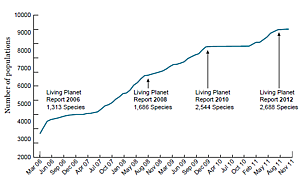Calculating the LPI | WWF

The LPI is based on population trends in over 2,600 vertebrate species worldwide.

Data on species’ populations from two or more years since 1970 are collected from a wide variety of published sources and entered into the LPI database.

In some cases, we have data on more than one population of a single species. For each population, the rate of change from one year to the next is calculated. If we have data from only a few, non-consecutive years, we assume there was a constant annual rate of change in the population between each data year.

Where we have data from many years (consecutive or not) we fit a curve through the data points using a statistical method called generalized additive modelling.

Where we have more than one population trend for a single species, the average rate of change across all of the populations is calculated for each year.

Then we calculate the average rate of change across all species from year to year. The index is set equal to 1 in 1970, and the average annual rate of population change is used to calculate the index value in each successive year.